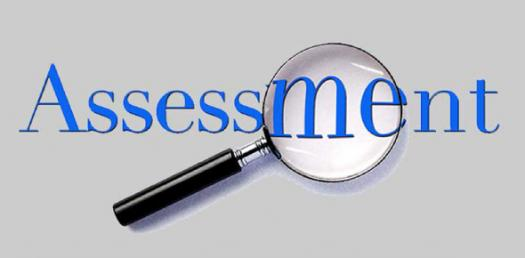10 Questions | Total Attempts: 552SettingsCreate your own QuizAre you in the second grade and are still trying to figure out mathematics with the addition and subtractions? Well don’t worry the easy questions below will help you have fun while practicing your addition skills. Remember math isn’t so hard it can be fun when you embrace it. Have fun!

• 1.
Give the correct answer.7 + 5 =
• A.

11

• B.

15

• C.

12

• D.

2

• 2.
Give the correct answer.25 + 15 =
• A.

10

• B.

40

• C.

50

• D.

35

• 3.
Fill in the blank.10 + ___ = 15
• A.

10

• B.

1

• C.

3

• D.

5

• 4.
Fill in the blank.3 + __ = 11
• A.

9

• B.

8

• C.

15

• D.

5

• 5.
Fill in the blank.7 + __ = 14
• A.

8

• B.

5

• C.

10

• D.

7

• 6.
Fill in the blank.2 + __ = 12
• A.

10

• B.

9

• C.

15

• D.

0

• 7.
Give the correct answer.15 + 15 =
• A.

35

• B.

40

• C.

50

• D.

30

• 8.
Give the correct answer.3 + 6 =
• A.

10

• B.

12

• C.

9

• D.

6

• 9.
Give the correct answer.24 + 0 =
• A.

25

• B.

24

• C.

0

• D.

10

• 10.
Fill in the blank.8 + __ = 16
• A.

9

• B.

10

• C.

8

• D.

25

Related TopicsBack to top
×

Wait!
Here's an interesting quiz for you.